# AKT-14/Tricolourability without Diagrams

Another place in which arcs of a diagram come up naturally is in the Wirtinger presentation for the fundamental group of the knot complement. Here is how the presentation is defined: each arc of the knot diagram corresponds with a generator, and each crossing corresponds with a relation between the generators of the incident arcs of the form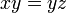$xy=yz$ where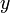$y$ is the generator corresponding with the overcrossing.
Now if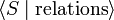$\langle S\mid \text{relations}\rangle$ is a Wirtinger presentation for a knot diagram, it's natural to think of a tricolouring as a map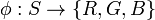$\phi: S \rightarrow \{R,G,B\}$. We'd like to try to extend this to a group homomorphism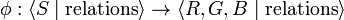$\phi:\langle S \mid \text{relations}\rangle \rightarrow \langle R,G,B \mid \text{relations} \rangle$. This works if target group has the relation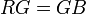$RG=GB$ along with all other relations obtained by permuting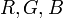$R,G,B$. These relations fix the target group as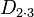$D_{2\cdot 3}$.
Thus, we've associated with each tricolouring a homomorphism from the fundamental group of the knot complement to$D_{2\cdot 3}$. Not every such homomorphism gives a tricolouring; for example, take the trivial homomorphism. I believe that the following is a sufficient condition for a homomorphism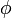$\phi$ to give a tricolouring: for every element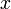$x$ in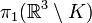$\pi_1(\R^3\setminus K)$ whose representative as a loop in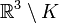$\mathbb{R}^3\setminus K$ has odd linking number with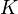$K$,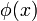$\phi(x)$ is an order 2 element in$D_{2\cdot 3}$. Hence, we can define tricolourings as certain kinds of homomorphisms from$\pi_1(\R^3\setminus K)$ to$D_{2\cdot 3}$ without having to choose a diagram.